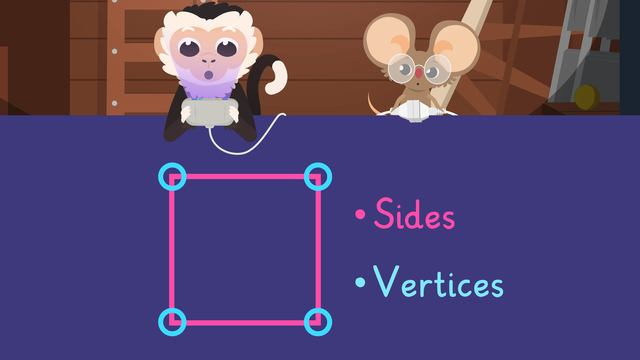# Sides and Vertices of ShapesRating

Ø 5.0 / 1 ratings

The authorsTeam Digital

## Basics on the topicSides and Vertices of Shapes

### In This Video

Nico and Nia discover a game that requires them to learn about sides and vertices so they can play it. They also learn about a vertex in geometry while looking at shapes in the game. After learning about 2D shapes sides and vertices, Nico gets a little too into his game, so much so that Nia has to take drastic action! At the bottom, you will find a sides and vertices worksheet.

### Vertices and Sides

Most 2D shapes have sides and vertices. So what are sides and vertices? A side is a straight line that makes up a closed 2D shape.A vertice, or vertex when we are talking about just one, is where two sides meet to form a corner.#### Square and Trapezoid Sides and Vertices

Squares and trapezoids are a type of quadrilateral. How many sides and vertices does a quadrilateral have? Quadrilaterals have 4 sides and 4 vertices, so when someone asks you what shape has 4 sides and 4 vertices, you can tell them all quadrilaterals do! Therefore, a square and trapezoid are examples of a shape with 4 sides and 4 vertices.#### Pentagon Sides and Vertices

How many sides and vertices does a pentagon have? A pentagon is a shape with 5 sides and 5 vertices, so when someone asks you what shape has 5 sides and 5 vertices, you can tell them pentagons do!#### Circle Sides and Vertices

How many sides and vertices does a circle have? A circle is a special case, because it has 0 sides and 0 vertices, so when someone asks you what shape has 0 sides and 0 vertices, you can tell them a circle!### Sides and Vertices of 2D Shapes - Review

Most shapes have sides and vertices. You can use these to help identify 2D shapes. A side is a straight line that makes up a closed 2D shape. A vertice, or vertex, is where two sides meet to form a corner.

Below you will find a 2D shapes sides and vertices worksheet.

### TranscriptSides and Vertices of Shapes

Nico has found an old game console in the attic. "Ohh, what is this?" "Hmm. I wonder?" It looks like a game has started on the console! However, Nico needs to learn about sides and vertices of 2D shapes to play the game. To help Nico play the game, let's learn about sides and vertices of 2D shapes. A 2D shape is a flat closed shape that almost always has sides and vertices. What are sides and vertices? Sides are the straight lines that form a shape, like all of these sides here. Vertices are the corners where two straight lines meet, like all of these corners here. If we talk about one corner, we call it a vertex. If we talk about more than one corner, we call them vertices. Knowing the number of sides and vertices a shape has can help you to identify shapes, or classify them into shape families! For example, this square has four sides and four vertices. A quadrilateral, like this square, always has four sides and four vertices! Now we have learned about sides and vertices, let's look at some different shapes. Here is a trapezoid. How many sides does it have? There are four sides. How many vertices does it have? There are four vertices. A trapezoid always has four sides and four vertices, which also makes it a part of the quadrilateral family. You might notice there are different shapes that share the same number of sides and vertices. Next we have a pentagon. How many sides and vertices does a pentagon have? There are five sides and five vertices! A pentagon always has five sides and five vertices. Some shapes are an exception to the rule that all 2D shapes have sides and vertices. One of them is this shape, which is a circle! What do you notice about the sides and vertices of this circle? There are zero sides and zero vertices. A circle will never have any sides or vertices. While Nico continues to play the Shape Mayhem game, let's review! Remember, a 2D shape almost always has sides and vertices. Sides are the straight lines that form a shape. Vertices are the corners where two straight lines meet. Some shapes are an exception to the rule that all 2D shapes have sides and vertices, and one of them is a circle. "Huh?" "I think that's enough game time for today, Nico!" "Oh, you're right! Let's do something else now!" "You were right Nia! Playing outside is much more fun!